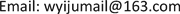﻿ 从约束优化问题的Lagrange对偶看《最优化方法》课程的驱动化教学 On the Driving Teaching of the Course of Optimizing Method from the Lagrange Duality of Constrained Optimizing Problem

Creative Education Studies
Vol. 07  No. 05 ( 2019 ), Article ID: 32453 , 5 pages
10.12677/CES.2019.75092

On the Driving Teaching of the Course of Optimizing Method from the Lagrange Duality of Constrained Optimizing Problem

Yiju Wang1, Naihua Xiu2

1School of Management Science, Qufu Normal Univeristy, Rizhao Shandong

2School of Science, Beijing Jiaotong Univeristy, BeijingReceived: Aug. 20th, 2019; accepted: Oct. 3rd, 2019; published: Oct. 10th, 2019ABSTRACT

The optimization theory, especially the Lagrange duality theory of constrained optimization problems, is an important and difficult part of the course Optimizing Method. In this regard, based on the optimality conditions of constrained optimization problems, we first introduce the definition of saddle point of constrained optimization problems, and then derive two bilevel extreme value problems related to constrained optimization problems, which leads to Lagrange dual programming of constrained optimization problems. In this way, Lagrange duality of constrained optimization problem is introduced layer by layer, which not only reduces the teaching difficulty of optimization duality theory, but also arouses students’ interest in learning.

Keywords:Optimizing Theory, Lagrange Duality, Driving Teaching

1曲阜师范大学管理学院，山东 日照

2北京交通大学理学院，北京1. 引言

2. Lagrange对偶规划问题的驱动化教学

$\begin{array}{l}\text{min}\text{ }\text{ }\text{ }\text{ }\text{ }\text{ }\text{ }\text{ }\text{ }\text{ }\text{ }\text{ }\text{ }\text{ }\text{ }\text{ }f\left(x\right)\\ \text{s}\text{.}\text{ }\text{t}\text{.}\text{ }\text{ }\text{ }\text{ }\text{ }\text{ }\text{ }\text{ }\text{ }\text{ }\text{ }\text{ }\text{ }\text{ }\text{ }\text{ }\text{ }\text{ }\text{ }\text{ }{c}_{i}\left(x\right)=0,\text{ }\text{ }\text{ }\text{ }\text{ }\text{ }\text{ }\text{ }\text{ }\text{ }i\in \mathcal{E}\end{array}$

$\nabla f\left({x}^{*}\right)=\underset{i\in \mathcal{E}}{\sum }{\lambda }_{\text{ }i}^{*}\nabla {c}_{i}\left({x}^{*}\right).$

$L\left(x,\lambda \right)=f\left(x\right)-\underset{i\in \mathcal{E}}{\sum }{\lambda }_{i}{c}_{i}\left(x\right).$

${\nabla }_{x}L\left({x}^{*},{\lambda }^{*}\right)=0.$

$\left\{\begin{array}{l}{\nabla }_{x}L\left({x}^{*},{\lambda }^{*}\right)=0,\\ {\nabla }_{\lambda }L\left({x}^{*},{\lambda }^{*}\right)=c\left({x}^{*}\right)=0.\end{array}$

$\begin{array}{l}\text{min}\text{ }\text{ }\text{ }\text{ }\text{ }\text{ }\text{ }\text{ }f\left(x\right)\\ \text{s}\text{.t}\text{.}\text{ }\text{ }\text{ }\text{ }\text{ }\text{ }\text{ }\text{ }\text{ }\text{ }\text{ }\text{ }\text{ }\text{ }{c}_{i}\left(x\right)=0,\text{ }\text{ }\text{ }\text{ }\text{ }i\in \mathcal{E}\\ \text{ }\text{ }\text{ }\text{ }\text{ }\text{ }\text{ }\text{ }\text{ }\text{ }\text{ }\text{ }\text{ }\text{ }\text{ }\text{ }\text{ }\text{ }\text{ }\text{ }\text{ }\text{ }\text{ }\text{ }{c}_{i}\left(x\right)\ge 0,\text{ }\text{ }\text{ }\text{ }\text{ }i\in \mathcal{I}\end{array}$

${s}^{\text{T}}\nabla {c}_{i}\left({x}^{*}\right)=0,\text{ }\text{ }\text{ }\text{ }i\in \mathcal{E},\text{ }\text{ }\text{ }\text{ }\text{ }\text{ }\text{ }\text{ }\text{ }\text{ }\text{ }\text{ }\text{ }\text{ }\text{ }{s}^{\text{T}}\nabla {c}_{i}\left({x}^{*}\right)>0,\text{ }\text{ }\text{ }\text{ }\text{ }\text{ }i\in \mathcal{I}\left({x}^{*}\right).$

$\left\{\begin{array}{l}\nabla f\left({x}^{*}\right)=\underset{i\in \mathcal{E}\cup \mathcal{I}}{\sum }{\lambda }_{\text{ }i}^{*}\nabla {c}_{i}\left({x}^{*}\right),\\ {c}_{i}\left({x}^{*}\right)\ge 0,\text{ }\text{ }\text{ }\text{ }\text{ }\text{ }\text{ }{\lambda }_{\text{ }i}^{*}\ge 0,\text{ }\text{ }\text{ }{\lambda }_{\text{ }i}^{*}{c}_{i}\left({x}^{*}\right)=0,\text{ }\text{ }\text{ }\text{ }i\in \mathcal{I}.\end{array}$

$\mathrm{min}\left\{f\left(x\right)|{c}_{i}\left(x\right)=0,i\in \mathcal{E};{c}_{i}\left(x\right)\ge 0,i\in \mathcal{I}\right\},$

$\left(x,{\lambda }^{*}\right)=f\left(x\right)-\underset{i\in \mathcal{E}\cup \mathcal{I}}{\sum }{\lambda }_{\text{ }i}^{*}{c}_{i}\left(x\right).$

$L\left({x}^{*},\lambda \right)\le L\left({x}^{*},{\lambda }^{*}\right)\le L\left(x,{\lambda }^{*}\right),\text{ }\forall {\lambda }_{\text{ }i}\ge 0,\text{\hspace{0.17em}}i\in \mathcal{I},\text{\hspace{0.17em}}x\in {R}^{n},$

$\left({x}^{*},{\lambda }^{*}\right)$ 称为该约束优化问题Lagrange函数的鞍点。

$\mathrm{min}\left\{f\left(x\right)|{c}_{i}\left(x\right)=0,i\in \mathcal{E};{c}_{i}\left(x\right)\ge 0,i\in \mathcal{I}\right\},$

$L\left(x,u,v\right)=f\left(x\right)-G{\left(x\right)}^{\text{T}}u-H{\left(x\right)}^{\text{T}}v,\text{ }x\in {R}^{n},\text{\hspace{0.17em}}u\in {R}_{+}^{|\mathcal{I}|},\text{\hspace{0.17em}}v\in {R}^{|\mathcal{E}|}.$

$L\left({x}^{*},u,v\right)\le L\left({x}^{*},{u}^{*},{v}^{*}\right)\le L\left(x,{u}^{*},{v}^{*}\right).$

$\underset{u\ge 0,v}{\mathrm{max}}\underset{x\in {R}^{n}}{\mathrm{min}}L\left(x,u,v\right)\text{ }\text{ }\underset{x\in {R}^{n}}{\mathrm{min}}\underset{u\ge 0,v}{\mathrm{max}}L\left(x,u,v\right)$

$\underset{u\ge 0,v}{\mathrm{sup}}L\left(x,u,v\right)=\left\{\begin{array}{l}\infty ,\text{ }\text{ }\text{\hspace{0.17em}}\text{ }\text{ }\text{ }若x\notin \Omega ,\\ f\left(x\right),\text{ }若x\in \Omega ,\end{array}$

$\underset{x\in {R}^{n}}{\mathrm{min}}\underset{u\ge 0,v}{\mathrm{max}}L\left(x,u,v\right)=\mathrm{min}\left\{f\left(x\right)|G\left(x\right)\ge 0,H\left(x\right)=0\right\}.$

$\theta \left(u,v\right)=\mathrm{min}\left\{L\left(x,u,v\right)|x\in {R}^{n}\right\}$

$\begin{array}{l}\mathrm{max}\text{ }\theta \left(u,v\right)\\ \text{s}\text{.t}\text{.}\text{ }u\in {R}_{+}^{|\mathcal{I}|},\text{\hspace{0.17em}}v\in {R}^{|\mathcal{E}|}\end{array}$

3. 结论

On the Driving Teaching of the Course of Optimizing Method from the Lagrange Duality of Constrained Optimizing Problem[J]. 创新教育研究, 2019, 07(05): 541-545. https://doi.org/10.12677/CES.2019.75092

1. 1. 陈宝林. 最优化理论与方法[M]. 北京: 清华大学出版社, 1989.

2. 2. 赵瑞安, 吴方. 非线性最优化理论与方法[M]. 杭州: 浙江科学技术出版社, 1992.

3. 3. 袁亚湘. 非线性最优化数值方法[M]. 上海: 上海科学技术出版社, 1993.

4. 4. 黄红选, 韩继业. 数学规划[M]. 北京: 清华大学出版社, 2006.

5. 5. 倪勤. 最优化方法与程序设计[M]. 北京: 科学出版社, 2009.

6. 6. 张立卫, 单锋. 最优化方法[M]. 北京: 科学出版社, 2010.

7. 7. 李董辉, 童小娇, 万中. 数值最优化算法与理论[M]. 北京: 科学出版社, 2010.

8. 8. 王宜举, 修乃华. 非线性最优化理论与方法[M]. 北京: 科学出版社, 2012.

9. 9. 黄正海, 苗新河. 最优化计算方法[M]. 北京: 科学出版社, 2015.

10. 10. Bazaraa, M.S., Sherali H.D. and Shetty C.M. (1993) Nonlinear Programming Theory and Algorithms. John Wiley and Sons, New York.

11. 11. Bertsekas, D.P. (1999) Nonlinear Programming. Athena Scientific, Belmont, MA.

12. 12. Nocedal, J. and Wright, S.J. (1999) Numerical Optimization. Springer Press, New York. https://doi.org/10.1007/b98874